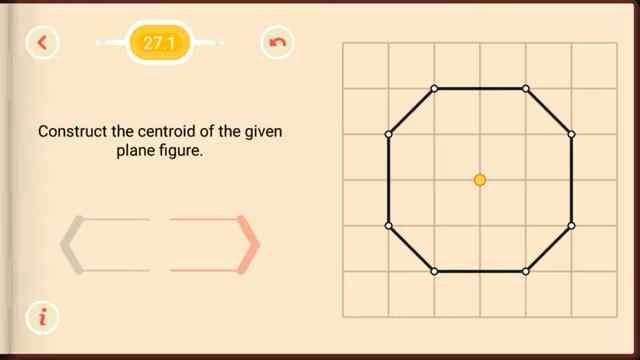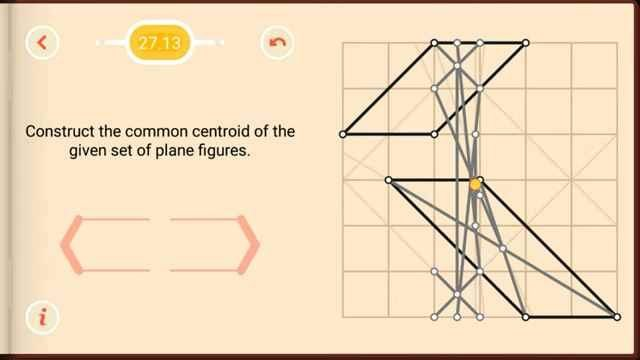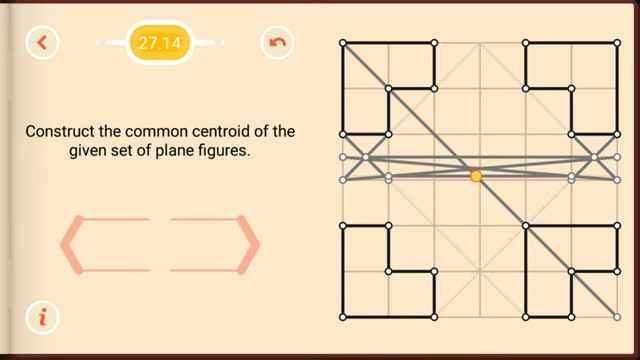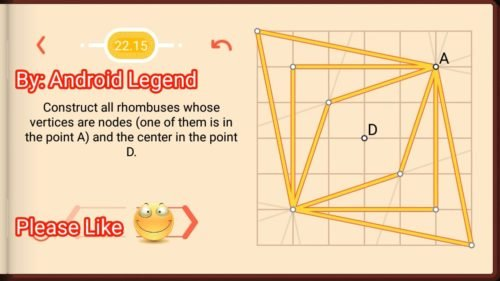# Pythagorea Centroids 27.1 27.2 27.3 27.4 27.5 27.6 27.7 27.8 27.9 27.10 27.11 27.12 27.13 27.14 27.15 27.16 27.17 SolutionPythagorea Centroid all levels solved here. Pythagorea is an android/iOS app developed by Horis International Limited. Solutions hints and answers to pythagorea are available in this post scroll down to find solutions to all the levels. This game is mostly focused on geometric puzzles and construction. The work space is divided into grids to draw lines. You should know all the basic Math operations. All lines and shapes are drawn on a grid whose cells are squares. Most of the game levels can be answered using natural intuition and by some basic laws of geometry.

If you are here for levels other than ‘Distance’ Go to directory of all other levels at : http://www.puzzlegamemaster.com/pythagorea-all-answers-solutions/

Pythagorea centroid 27.1 Solution:Pythagorea centroid 27.2 Solution:Pythagorea centroid 27.3 Solution:Pythagorea centroid 27.4 Solution:Pythagorea centroid 27.5 Solution:Pythagorea centroid 27.6 Solution:Pythagorea centroid 27.7 Solution:Pythagorea centroid 27.8 Solution:Pythagorea centroid 27.9 Solution:Pythagorea centroid 27.10 Solution:Pythagorea centroid 27.11 Solution:Pythagorea centroid 27.12 Solution:Pythagorea centroid 27.13 Solution:Pythagorea centroid 27.14 Solution:Pythagorea centroid 27.15 Solution:Pythagorea centroid 27.16 Solution:Pythagorea centroid 27.17 Solution:Thanks for visiting, If you have any doubts regarding pythagorea, You can comment below.

# Pythagorea Rhombuses Level 22.17 Solution/AnswerPythagorea Rhombuses Level 22.17 New Version Game Answers,  detailed solutions, Tips, and Walkthrough. Scroll below to find answer to this level.

Pythagorea is android/iOS app developed by Horis International Limited. Solutions hints and answers to pythagorea are available in this post scroll down to find solutions to all the levels.

This game is mostly focused on geometric puzzles and construction. The work space is divided into grids to draw lines. You should know all the basic Math operations. All lines and shapes are drawn on a grid whose cells are squares. Most of the game levels can be answered using natural intuition and by some basic laws of geometry.• Pythagorea Level 22.17: Inscribe a rhombus in the triangle so that they share the common angle A.If you have any doubt regarding Pythagorea answers given here you can watch video below or you can comment on this post-

# Pythagorea Rhombuses Level 22.16 Solution/AnswerPythagorea Rhombuses Level 22.16 New Version Game Answers,  detailed solutions, Tips, and Walkthrough. Scroll below to find answer to this level.

Pythagorea is android/iOS app developed by Horis International Limited. Solutions hints and answers to pythagorea are available in this post scroll down to find solutions to all the levels.

This game is mostly focused on geometric puzzles and construction. The work space is divided into grids to draw lines. You should know all the basic Math operations. All lines and shapes are drawn on a grid whose cells are squares. Most of the game levels can be answered using natural intuition and by some basic laws of geometry.• Pythagorea Level 22.16: Inscribe a rhombus in the triangle so that they share the common angle A.If you have any doubt regarding Pythagorea answers given here you can watch video below or you can comment on this post-

# Pythagorea Rhombuses Level 22.15 Solution/AnswerPythagorea Rhombuses Level 22.15 New Version Game Answers,  detailed solutions, Tips, and Walkthrough. Scroll below to find answer to this level.

Pythagorea is android/iOS app developed by Horis International Limited. Solutions hints and answers to pythagorea are available in this post scroll down to find solutions to all the levels.

This game is mostly focused on geometric puzzles and construction. The work space is divided into grids to draw lines. You should know all the basic Math operations. All lines and shapes are drawn on a grid whose cells are squares. Most of the game levels can be answered using natural intuition and by some basic laws of geometry.Pythagorea Level 22.15: Construct all rhombuses whose vertices are nodes (one of them is in the point A) and the center in the point D.If you have any doubt regarding Pythagorea answers given here you can watch video below or you can comment on this post-

# Pythagorea Rhombuses Level 22.14 Solution/AnswerPythagorea Rhombuses Level 22.14 New Version Game Answers,  detailed solutions, Tips, and Walkthrough. Scroll below to find answer to this level.

Pythagorea is android/iOS app developed by Horis International Limited. Solutions hints and answers to pythagorea are available in this post scroll down to find solutions to all the levels.

This game is mostly focused on geometric puzzles and construction. The work space is divided into grids to draw lines. You should know all the basic Math operations. All lines and shapes are drawn on a grid whose cells are squares. Most of the game levels can be answered using natural intuition and by some basic laws of geometry.• Pythagorea Level 22.14: Construct a rhombus whose Vertices are four of the given points.If you have any doubt regarding Pythagorea answers given here you can watch video below or you can comment on this post-

# Pythagorea Rhombuses Level 22.13 Solution/AnswerPythagorea Rhombuses Level 22.13 New Version Game Answers,  detailed solutions, Tips, and Walkthrough. Scroll below to find answer to this level.

Pythagorea is android/iOS app developed by Horis International Limited. Solutions hints and answers to pythagorea are available in this post scroll down to find solutions to all the levels.

This game is mostly focused on geometric puzzles and construction. The work space is divided into grids to draw lines. You should know all the basic Math operations. All lines and shapes are drawn on a grid whose cells are squares. Most of the game levels can be answered using natural intuition and by some basic laws of geometry.• Pythagorea Level 22.13: Inscribe a rhombus in the triangle so that they share the common angle A.If you have any doubt regarding Pythagorea answers given here you can watch video below or you can comment on this post-

# Pythagorea Rhombuses Level 22.12 Solution/AnswerPythagorea Rhombuses Level 22.12 New Version Game Answers,  detailed solutions, Tips, and Walkthrough. Scroll below to find answer to this level.

Pythagorea is android/iOS app developed by Horis International Limited. Solutions hints and answers to pythagorea are available in this post scroll down to find solutions to all the levels.

This game is mostly focused on geometric puzzles and construction. The work space is divided into grids to draw lines. You should know all the basic Math operations. All lines and shapes are drawn on a grid whose cells are squares. Most of the game levels can be answered using natural intuition and by some basic laws of geometry.• Pythagorea Level 22.12: Inscribe a rhombus in the triangle so that they share the common angle A.If you have any doubt regarding Pythagorea answers given here you can watch video below or you can comment on this post-

# Pythagorea Rhombuses Level 22.11 Solution/AnswerPythagorea Rhombuses Level 22.11 New Version Game Answers,  detailed solutions, Tips, and Walkthrough. Scroll below to find answer to this level.

Pythagorea is android/iOS app developed by Horis International Limited. Solutions hints and answers to pythagorea are available in this post scroll down to find solutions to all the levels.

This game is mostly focused on geometric puzzles and construction. The work space is divided into grids to draw lines. You should know all the basic Math operations. All lines and shapes are drawn on a grid whose cells are squares. Most of the game levels can be answered using natural intuition and by some basic laws of geometry.• Pythagorea Level 22.11: Construct all rhombuses (not squares) whose vertices are nodes (one of them is in the point A) and whose side is of length root 5.If you have any doubt regarding Pythagorea answers given here you can watch video below or you can comment on this post-

# Pythagorea Rhombuses Level 22.10 Solution/AnswerPythagorea Rhombuses Level 22.10 New Version Game Answers,  detailed solutions, Tips, and Walkthrough. Scroll below to find answer to this level.

Pythagorea is android/iOS app developed by Horis International Limited. Solutions hints and answers to pythagorea are available in this post scroll down to find solutions to all the levels.

This game is mostly focused on geometric puzzles and construction. The work space is divided into grids to draw lines. You should know all the basic Math operations. All lines and shapes are drawn on a grid whose cells are squares. Most of the game levels can be answered using natural intuition and by some basic laws of geometry.• Pythagorea Level 22.10: Inscribe a rhombus in a given parallelogram so that they have the same center. One of its vertices is in the point A.If you have any doubt regarding Pythagorea answers given here you can watch video below or you can comment on this post-

# Pythagorea Rhombuses Level 22.9 Solution/AnswerPythagorea Rhombuses Level 22.9 New Version Game Answers,  detailed solutions, Tips, and Walkthrough. Scroll below to find answer to this level.

Pythagorea is android/iOS app developed by Horis International Limited. Solutions hints and answers to pythagorea are available in this post scroll down to find solutions to all the levels.

This game is mostly focused on geometric puzzles and construction. The work space is divided into grids to draw lines. You should know all the basic Math operations. All lines and shapes are drawn on a grid whose cells are squares. Most of the game levels can be answered using natural intuition and by some basic laws of geometry.• Pythagorea Level 22.9: Inscribe a rhombus in a given parallelogram so that they have the same center. One of its vertices is in the point A.If you have any doubt regarding Pythagorea answers given here you can watch video below or you can comment on this post-